Home    |    Teacher    |    Parents    |    Glossary    |    About UsFinding the square root of a number is the inverse operation of squaring that number. Remember, the square of a number is that number times itself.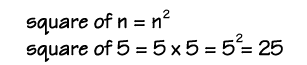The perfect squares are the squares of the whole numbers.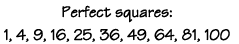The square root of a number, n, written below is the number that gives n when multiplied by itself.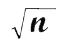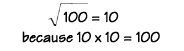Homework Help | Pre-Algebra | NumbersEmail this page to a friendSearch·  Place value·  Decimal numbers·  Estimating and     rounding·  Adding / subtracting     decimals·  Multiplying decimals·  Dividing decimals·  Percent·  Exponents·  Square roots·  Signed integers·  Adding and     subtracting integers·  Multiplying and     dividing integers·  Properties of integers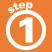First Glance In Depth Examples WorkoutSquare roots